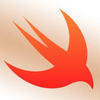# I dont know where my problem is !

Pls tell me where is the problem

fizzBuzz.swift
```func fizzBuzz(n: Int) -> String {
if n % 6 == 0 {
return "Fizz"
} else if n % 6 == 0 {
return "Buzz"
}else if n%5 == 0 && n%5 == 0 {
return "FizzBuzz"
} else {
return""
}
return "\(n)"
}
```Hi Michael - After looking at your code further, there are several issues. You are not checking the correct inputs (I would go back and watch the instructions video). To answer your question on this one though you need to check and return 'Fizzbuzz" first before you can check the other variables

```func fizzBuzz(n: Int) -> String {
// Enter your code between the two comment markers
if (n % 3 == 0) && (n % 5 == 0) {
return("FizzBuzz")
} else if (n % 3 == 0) {
return ("Fizz")
} else if (n % 5 == 0) {
return("Buzz")
} else {
return("n")
}
// End code
}
```Hi Michael -

This is a common mistake that is made on the particular challenge as it is asked in some coding interviews. You need to return "FizzBuzz" first, you should be able to figure it out from there

HI Jeff , so I return FizzBuzz but from where ? else { return"FizzBuzz" } Like this ?